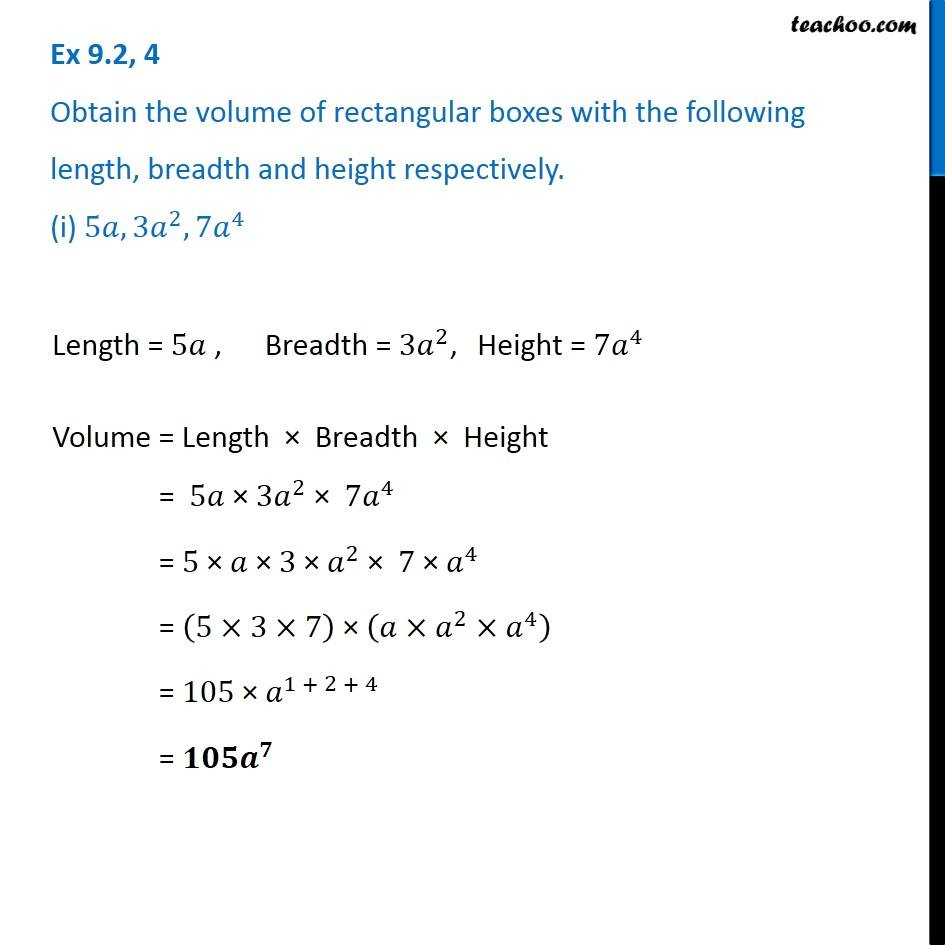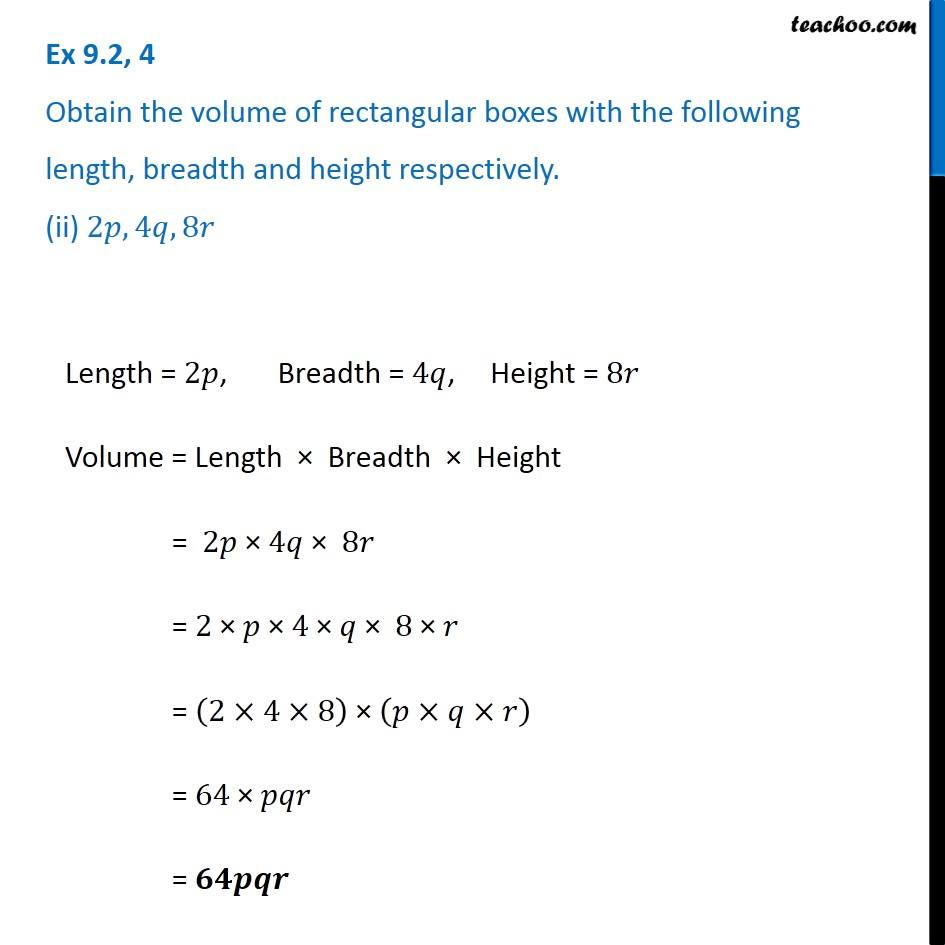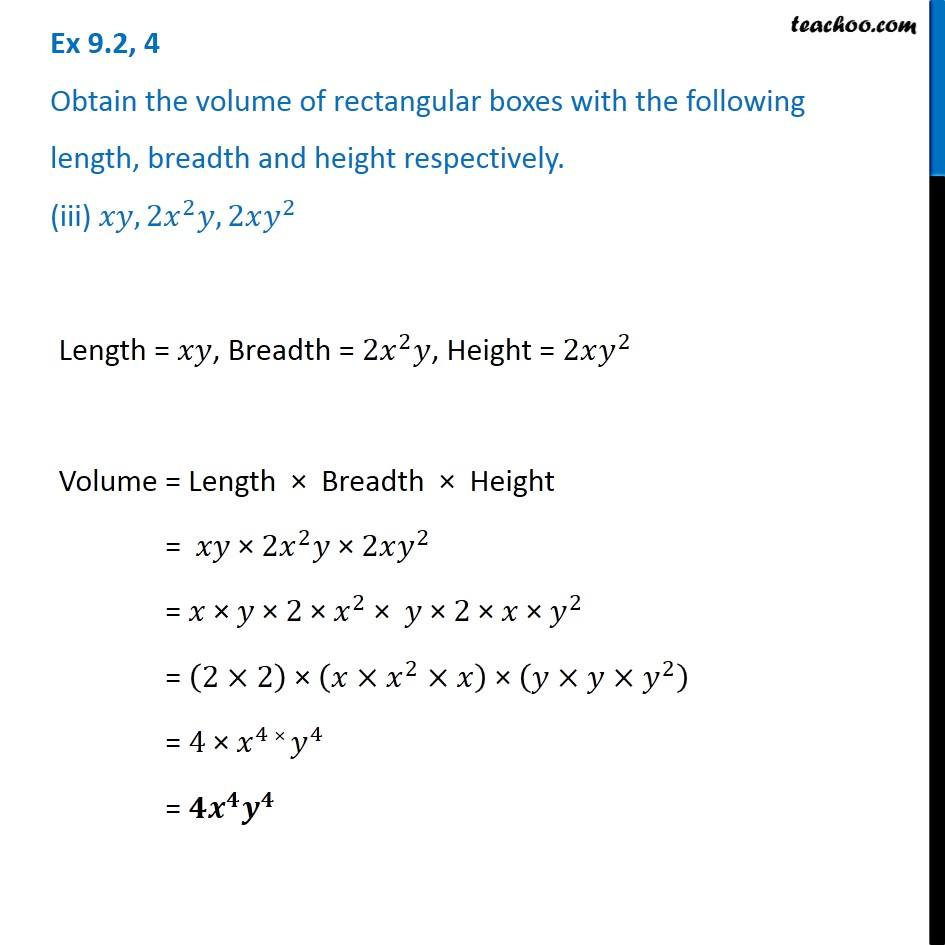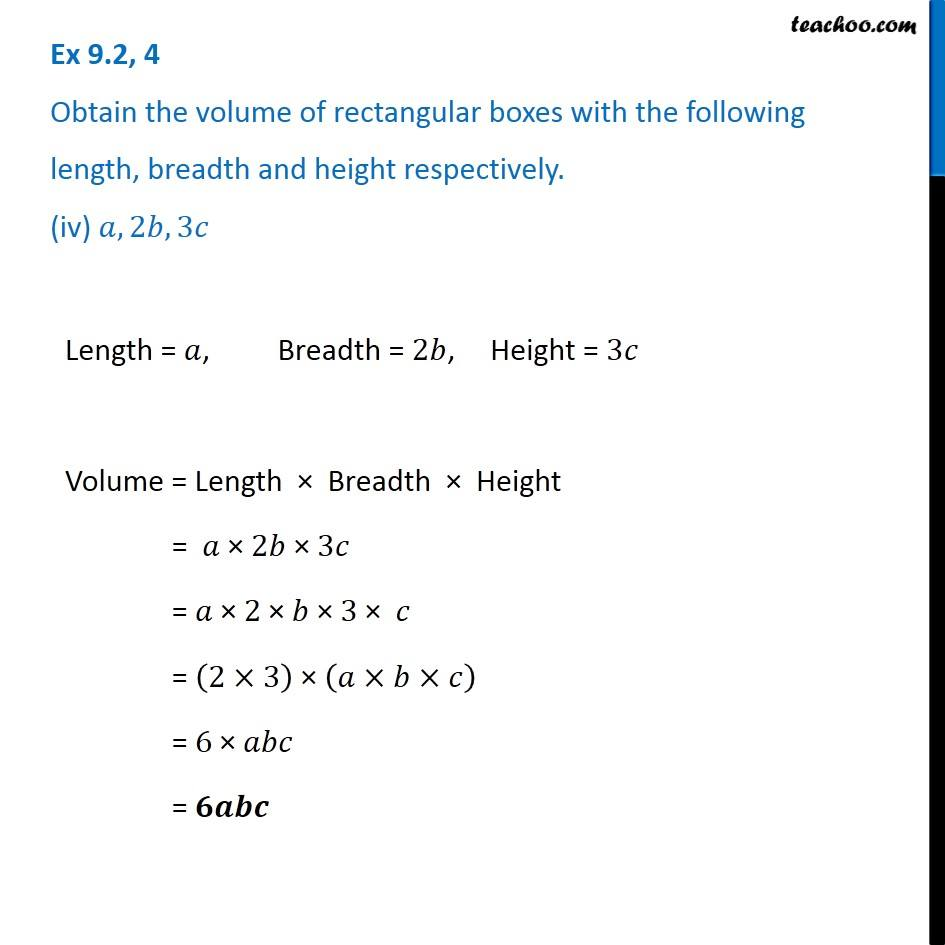1. Chapter 9 Class 8 Algebraic Expressions and Identities
2. Serial order wise
3. Ex 9.2

Transcript

Ex 9.2, 4 Obtain the volume of rectangular boxes with the following length, breadth and height respectively. (i) 5𝑎, 3𝑎^2, 7𝑎^4 Length = 5𝑎 , Breadth = 〖3𝑎〗^2, Height = 〖7𝑎〗^4 Volume = Length × Breadth × Height = 5𝑎 × 〖3𝑎〗^2 × 〖7𝑎〗^4 = 5 × 𝑎 × 3 × 𝑎^2 × 7 × 𝑎^4 = (5×3×7) × (𝑎×𝑎^2×𝑎^4 ) = 105 × 𝑎^(1 + 2 + 4) = 𝟏𝟎𝟓𝒂^𝟕 Ex 9.2, 4 Obtain the volume of rectangular boxes with the following length, breadth and height respectively. (ii) 2𝑝, 4𝑞, 8𝑟 Length = 2𝑝, Breadth = 4𝑞, Height = 8𝑟 Volume = Length × Breadth × Height = 2𝑝 × 4𝑞 × 8𝑟 = 2 × 𝑝 × 4 × 𝑞 × 8 × 𝑟 = (2×4×8) × (𝑝×𝑞×𝑟) = 64 × 𝑝𝑞𝑟 = 𝟔𝟒𝒑𝒒𝒓 Ex 9.2, 4 Obtain the volume of rectangular boxes with the following length, breadth and height respectively. (iii) 𝑥𝑦, 2𝑥^2 𝑦, 2𝑥𝑦^2 Length = 𝑥𝑦, Breadth = 2𝑥^2 𝑦, Height = 2𝑥𝑦^2 Volume = Length × Breadth × Height = 𝑥𝑦 × 2𝑥^2 𝑦 × 2𝑥𝑦^2 = 𝑥 × 𝑦 × 2 × 𝑥^2 × 𝑦 × 2 × 𝑥 × 𝑦^2 = (2×2) × (𝑥×𝑥^2×𝑥) × (𝑦×𝑦×𝑦^2 ) = 4 × 𝑥^4 × 𝑦^4 = 𝟒𝒙^𝟒 𝒚^𝟒 Ex 9.2, 4 Obtain the volume of rectangular boxes with the following length, breadth and height respectively. (iv) 𝑎, 2𝑏, 3𝑐 Length = 𝑎, Breadth = 2𝑏, Height = 3𝑐 Volume = Length × Breadth × Height = 𝑎 × 2𝑏 × 3𝑐 = 𝑎 × 2 × 𝑏 × 3 × 𝑐 = (2×3) × (𝑎×𝑏×𝑐) = 6 × 𝑎𝑏𝑐 = 𝟔𝒂𝒃𝒄

Ex 9.2

About the AuthorDavneet Singh
Davneet Singh is a graduate from Indian Institute of Technology, Kanpur. He has been teaching from the past 9 years. He provides courses for Maths and Science at Teachoo.